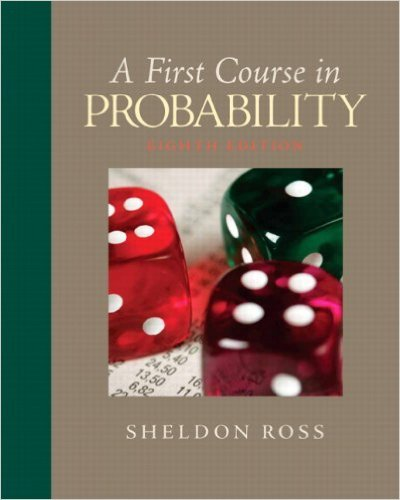×
×

# An instructor gives her class a set of 10 problemswith theISBN: 9780136033134 163

## Solution for problem 37 Chapter 2

First Course in Probability | 8th Edition

• Textbook Solutions
• 2901 Step-by-step solutions solved by professors and subject experts
• Get 24/7 help from StudySoup virtual teaching assistantsFirst Course in Probability | 8th Edition

4 5 1 356 Reviews
17
5
Problem 37

An instructor gives her class a set of 10 problemswith the information that the final exam will consistof a random selection of 5 of them. If a studenthas figured out how to do 7 of the problems, what isthe probability that he or she will answer correctly(a) all 5 problems?(b) at least 4 of the problems?

Step-by-Step Solution:
Step 1 of 3

Review of Notes 4: i = 1, 2 ….. n j = 1, 2 ….r i ̅ ∑ ∑ ̅ ∑ ∑ ∑ ∑ ( ̅) => Overall Variation (SST total sum of squares) ∑ ∑ ( ) = {( ̅ ) ̅ ̅ } = ∑ ∑ ( ̅ ) ∑ ∑...

Step 2 of 3

Step 3 of 3

##### ISBN: 9780136033134

This full solution covers the following key subjects: Answer, class, consist, correctly, exam. This expansive textbook survival guide covers 10 chapters, and 495 solutions. The full step-by-step solution to problem: 37 from chapter: 2 was answered by , our top Statistics solution expert on 11/23/17, 05:06AM. The answer to “An instructor gives her class a set of 10 problemswith the information that the final exam will consistof a random selection of 5 of them. If a studenthas figured out how to do 7 of the problems, what isthe probability that he or she will answer correctly(a) all 5 problems?(b) at least 4 of the problems?” is broken down into a number of easy to follow steps, and 56 words. Since the solution to 37 from 2 chapter was answered, more than 232 students have viewed the full step-by-step answer. This textbook survival guide was created for the textbook: First Course in Probability, edition: 8. First Course in Probability was written by and is associated to the ISBN: 9780136033134.

Unlock Textbook Solution